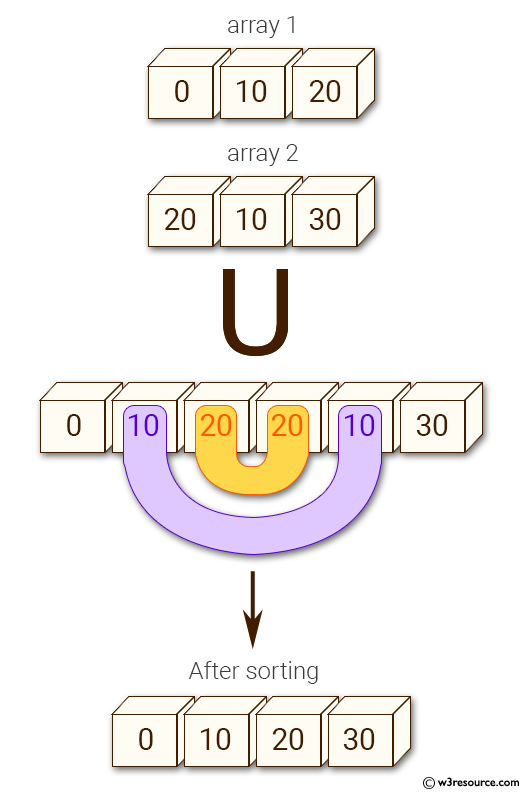﻿ NumPy: Find the union of two arrays - w3resource# NumPy: Find the union of two arrays

## NumPy: Array Object Exercise-22 with Solution

Write a NumPy program to find the union of two arrays. Union will return the unique, sorted array of values that are in either of the two input arrays.

Pictorial Presentation:Sample Solution:

Python Code:

``````import numpy as np
array1 = np.array([0, 10, 20, 40, 60, 80])
print("Array1: ",array1)
array2 = [10, 30, 40, 50, 70]
print("Array2: ",array2)
print("Unique sorted array of values that are in either of the two input arrays:")
print(np.union1d(array1, array2))
```
```

Sample Output:

```Array1:  [ 0 10 20 40 60 80]
Array2:  [10, 30, 40, 50, 70]
Unique sorted array of values that are in either of the two input array
s:
[ 0 10 20 30 40 50 60 70 80]
```

Python Code Editor:

Have another way to solve this solution? Contribute your code (and comments) through Disqus.

What is the difficulty level of this exercise?

Test your Python skills with w3resource's quiz

﻿

## Python: Tips of the Day

Set comprehension:

```>>> m = {x ** 2 for x in range(5)}
>>> m
{0, 1, 4, 9, 16}
```